# Splitting field of a polynomial

(diff) ← Older revision | Latest revision (diff) | Newer revision → (diff)

The smallest field containing all roots of that polynomial. More exactly, an extensionof a fieldis called the splitting field of a polynomialover the fieldifdecomposes overinto linear factors: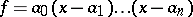and if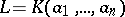(see Extension of a field). A splitting field exists for any polynomial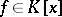, and it is defined uniquely up to an isomorphism that is the identity on. It follows from the definition that a splitting field is a finite algebraic extension of.

Examples. The field of complex numbersserves as the splitting field of the polynomial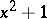over the fieldof real numbers. Any finite field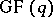, where, is the splitting field of the polynomial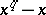over the prime subfield.